## TiNSpire CX: Solve System of Differential Equations using LaPlace Transform – Step by Step

Say you have to solve the system of Differential Equations shown in below’s image. Launch the Differential Equations Made Easy app (download at www.TiNspireApps.com) , go to Laplace Transforms in the menu and just type in as shown below:

Scrolling down to view all steps finally shows the correct final answer:

Posted on Categories differential equation, laplace transform

## TiNspire CX CAS : Solve Differential Equations – Step by Step – using LaPlace Transforms

Using the Differential Equations Made Easy APP at https://tinspireapps.com/?a=deqme you can solve Differential Equations using LaPlace Transforms as shown below:

Posted on Categories differential equation, laplace transform

## TiNspire: LaPlace Transforms of a Piecewise-Defined Function

Laplace transform over Piecewise def. Function

Example:

f(1) = 3 defined over 0<= t <2

f(2) = t  defined over t >= 2

To find the LaPlace Transform use Differential Equations Made Easy at

https://www.tinspireapps.com/?a=DEQME  and select LAPLACE TRANSFORM OF PIECEWISE DEFINED FUNCTION and enter as follows: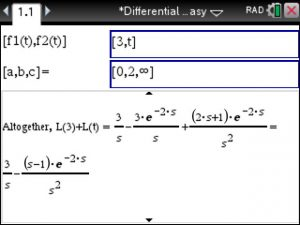We use infinity since the function f2 is not bounded. If it was bounded by for example 10 then we would have entered as [0,2,10]

Posted on Categories differential equation, laplace transform

## Laplace Transforms and Inverse using the TiNspire CX – Step by Step

Below find a bunch of Laplace and Inverse Laplace Transform examples

using the TiNspire CX CAS  and Differential Equations Made Easy at

Here we are using the Integral definition of the Laplace Transform to find solutions.

It takes a TiNspire CX CAS to perform those integrations.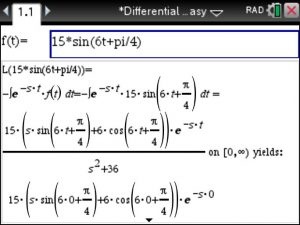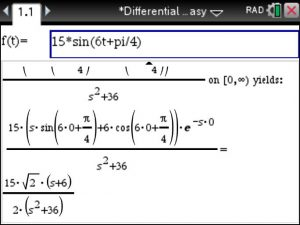Examples of Inverse Laplace Transforms, again using Integration: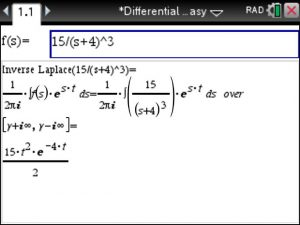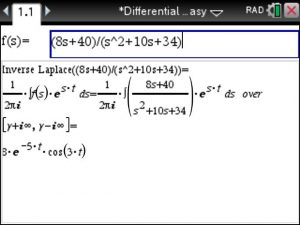Posted on Categories differential equation, laplace transform

## Laplace Transform of the Dirac Delta Function using the TiNspire Calculator

To find the Laplace Transform of the Dirac Delta Function just selectNext enter the c value and view the Laplace transform below the entry box.Posted on Categories laplace transform

## LaPlace Transforms involving Unit Step and Heavyside Functions using the TiNspire CAS

The Differential Equation Made Easy Made Easy for the TiNspire at www.TiNspireApps.com should be called Transforms Made Easy as we include a lot of LaPlace Transform options involving Unit Step and Heavyside Functions.

Check out the screenshot of the menu below:The Laplace Transform of a Unit Step function is performed by entering the shift c :To LaPlace Transform Unit Step functions of the type u(t-c)*f(t-c) you just enter
f(t) and again the shift c . Voila.To LaPlace Transform Unit Step functions of the type u(t-c)*f(t) you just enter
f(t) and again the shift c  and just follow the steps provided.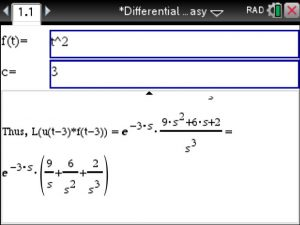This and many other APPS available at www.TiNspireApps.com

Posted on

## Finding Transforms using the TiNspire CX CAS: Fourier, Laplace and Z Transforms – using Differential Equations Made Easy

Finding Transforms using the TiNspire is pretty straightforward. Using the Differential Equation Made Easy APP you can do the following:
1) LaPlace and Inverse LaPlace Transforms
View some examples here:
https://tinspireapps.com/blog/simple-inverse-laplace-transforms-using-the-ti-nspire-cas-cx/

2) Fourier and Inverse Fourier Transforms
3) Fourier Series
4) Z Transforms
5) Inverse Z Transforms
( Z Transforms using the TiNspire can viewed here https://tinspireapps.com/blog/z-transforms-using-the-tinspire-cx-cas/ )

You can also look up the following Transforms as reference tables:

LaPlace and Inverse LaPlace Transforms
Fourier and Inverse Fourier Transforms
Z Transforms and Inverse Z Transforms

Below’s screenshot gives an idea of the Transforms and its uses.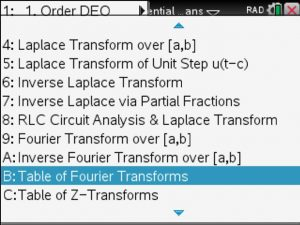Additionally, 2. order Differential Equations can be solved using LaPlace Transforms – step by step.

Posted on

## Advanced Inverse Laplace Transforms (Partial Fractions, Poles, Residues) using the TiNspire CAS CX – in Differential Equations Made Easy

See how to find Advanced Inverse Laplace Transforms  involving Partial Fractions, Poles, Residues using the TiNspire CAS CX in the Differential Equations Made Easy APP: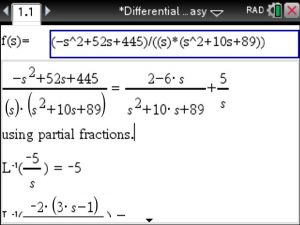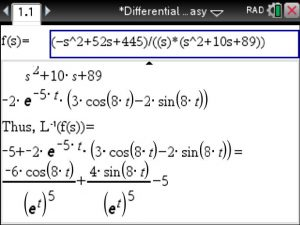Another example:Posted on

## Customer lost test anxiety using Made Easy APPS : Laplace, Fourier Transforms with Steps

Customer Testimonial :

Basically, I always struggled with calculus so those steps are essential…

Laplace, Fourier Transforms with Steps have made my life much easier because I can check my errors and I no longer have test anxiety…

The book is sometimes too complicated to understand with all the formulas and variables…

I took linear algebra and got a D and because of your apps I will retake it with all my pearly white showing…#IEEE

## Step by Step Engineering Mathematics using the Ti-NSpire CAS CX calculator program

Attention Engineers with a TI-Nspire CAS CX :

Engineering Mathematics has become much easier : this Step by Step Ti-nspire app covers Math Topics for Engineers (i.e. FE Exam) such as Algebra, Complex Numbers, Conics, Trigonometry, Exponential and Logarithmic Functions, Calculus, Differential Equations with LaPlace Transforms, Statistics, Probability, Combinations & Permutations, Matrices and Vectors. Read Definitions & Theories.  For more details visit: http://www.tinspireapps.com/?a=EMME

Posted on